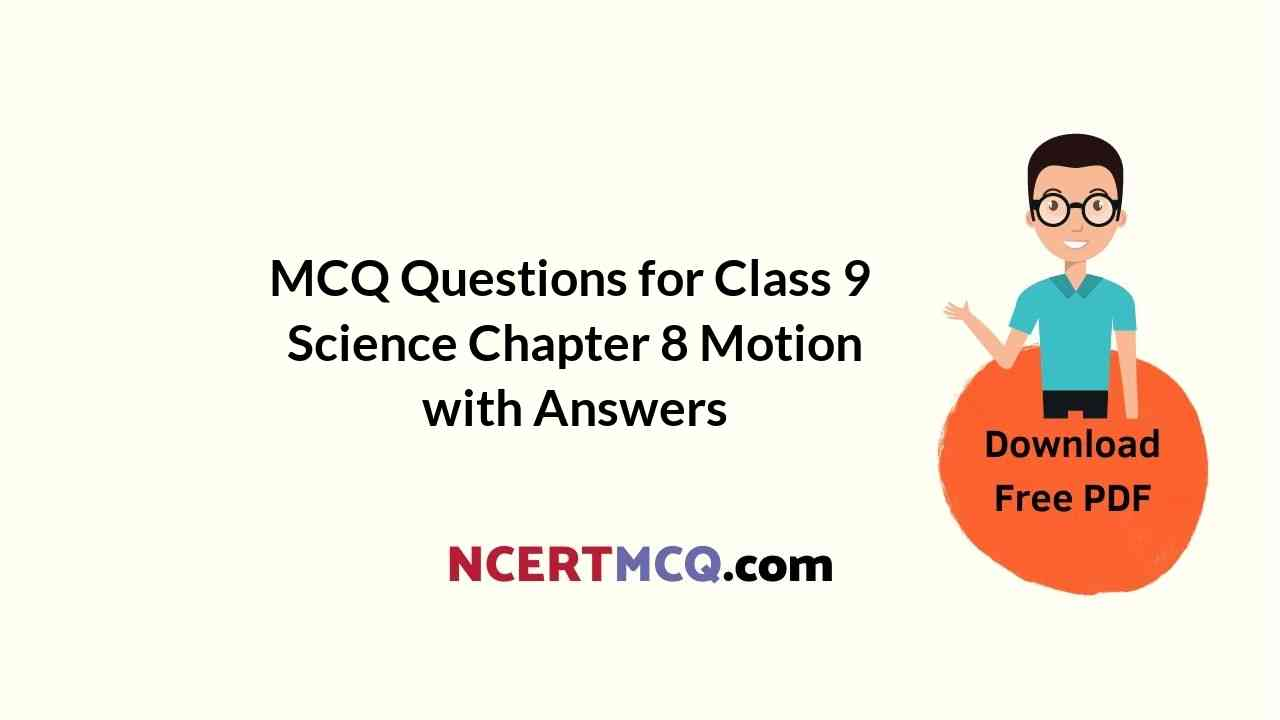Check the below NCERT MCQ Questions for Class 9 Science Chapter 8 Motion with Answers Pdf free download. MCQ Questions for Class 9 Science with Answers were prepared based on the latest exam pattern. We have Provided Motion Class 9 Science MCQs Questions with Answers to help students understand the concept very well. https://ncertmcq.com/mcq-questions-for-class-9-science-with-answers/

You can refer to Online Education NCERT Solutions for Class 9 Science Chapter 9 Motion to revise the concepts in the syllabus effectively and improve your chances of securing high marks in your board exams.

## Motion Class 9 MCQs Questions with Answers

This Displacement Calculator finds the displacement (distance traveled) by an object using its initial and final velocities as well as the time traveled.

Motion Class 9 MCQ Chapter 8 Question 1.
A particle is moving in a circular path of radius r. The displacement after half a circle would be:
(a) Zero
(b) πr
(c) 2r
(d) 2πr

Motion MCQ Class 9 Chapter 8 Question 2.
A body is thrown vertically upward with velocity u, the greatest height h to which it will rise is,
(a) ulg
(b) u2l2g
(c) u2lg
(d) ul2g

Class 9 Motion MCQ Chapter 8 Question 3.
The numerical ratio of displacement to distance for a moving object is
(a) always less than 1
(b) always equal to 1
(c) always more than 1
(d) equal or less than 1

Answer: (d) equal or less than 1

This is a free online acceleration calculator. enter velocity and time value then click on calculate and the result will be instant displayed

Class 9 Science Chapter 8 MCQ Question 4.
If the displacement of an object is proportional to square of time, then the object moves with
(a) uniform velocity
(b) uniform acceleration
(c) increasing acceleration
(d) decreasing acceleration

MCQ Of Motion Class 9 Chapter 8 Question 5.
From the given υ – t graph, it can be inferred that the object is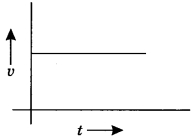(a) in uniform motion
(b) at rest
(c) in non-uniform motion
(d) moving with uniform acceleration

Physics MCQ Questions Class 9 Motion With Answers Question 6.
Suppose a boy is enjoying a ride on a merry-go-round which is moving with a constant speed of 10 ms-1 It implies that the boy is
(a) at rest
(b) moving with no acceleration
(c) in accelerated motion
(d) moving with uniform velocity

MCQ On Motion Class 9 Chapter 8 Question 7.
Area under a υ -1 graph represents a physical quantity which has the unit
(а) m2
(b) m
(c) m3
(d) ms-1

Motion MCQ Chapter 8 Question 8.
Four cars A, B, C and D are moving on a levelled road. Their distance versus time graphs are shown in the adjacent figure. Choose the correct statement.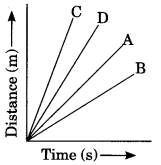(a) Car A is faster than car D.
(b) Car B is the slowest.
(c) Car D is faster than car C.
(d) Car C is the slowest.

Answer: (b) Car B is the slowest.

Class 9 Science Ch 8 MCQ Question 9.
Which of the following figures correctly represents uniform motion of a moving object?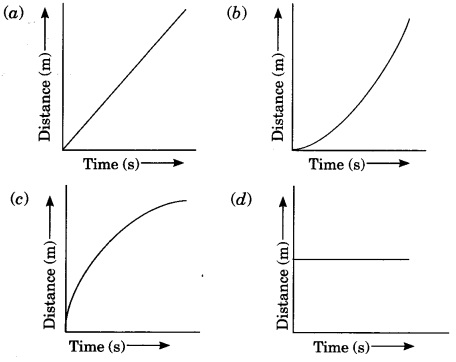Ch 8 Science Class 9 MCQ Question 10.
Slope of a velocity-time graph gives
(a) the distance
(b) the displacement
(c) the acceleration
(d) the speed

Motion Chapter Class 9 MCQ Question 11.
In which of the following cases of motions, the distance moved and the magnitude of displacement are equal?
(a) If the car is moving on a straight road
(b) If the car is moving in Circular path
(c) The pendulum is moving to and fro
(d) The earth is revolving around the sun.

Class 9 Science Motion MCQ Question 12.
A boy goes from A to B with a velocity of 20 m/min and comes back from B to A with a velocity of 30 m/min. The average velocity of the boy during the whole journey is
(a) 24 m/min
(b) 25 m/s
(c) Zero
(d) 20 m/minMotion Class 9 MCQ With Answers Pdf Question 13.
Velocity-time graph of an object is given below. The object has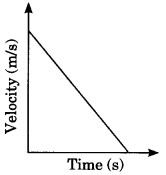(a) Uniform velocity
(b) Uniform speed
(c) Uniform retardation
(d) Variable acceleration

Class 9 Chapter 8 Science MCQ Question 14.
Which one of the following graphs shows the object to be stationary?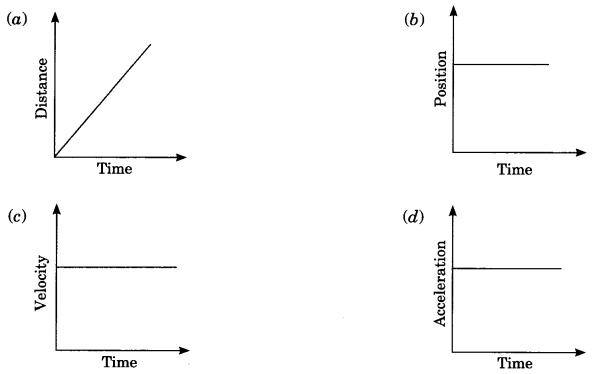Motion Class 9 MCQ With Answers Question 15.
A body is projected vertically upward from the ground. Taking vertical upward direction as positive and point of projection as origin, the sign of displacement of the body from the origin when it is at height h during upward and downward journey will be
(a) Positive, positive
(b) Positive, negative
(c) Negative, negative
(d) Negative, positive

Class 9 Physics Motion MCQ Question 16.
According to the given velocity-time graph, the object(a) is moving with uniform velocity
(b) has some initial velocity
(c) is moving uniformly with some initial velocity
(d) is at rest

Answer: (b) has some initial velocity

Question 17.
The ratio of speed to the magnitude of velocity when the body is moving in one direction is
(a) Less than one
(b) Greater than one
(c) Equal to one
(d) Greater than or equal to one

Question 18.
A car is moving along a straight road with uniform velocity. It is shown in the graph.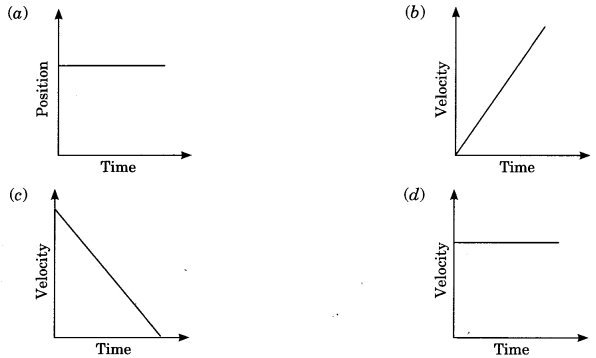Question 19.
Which of the following situations is possible?
(a) An object can have acceleration, but constant velocity.
(b) The velocity of an object may be zero but acceleration is not zero.
(c) Distance and the magnitude of displacement are equal in circular motion.
(d) Average speed and the magnitude of average velocity are always equal in circular motion.

Answer: (b) The velocity of an object may be zero but acceleration is not zero.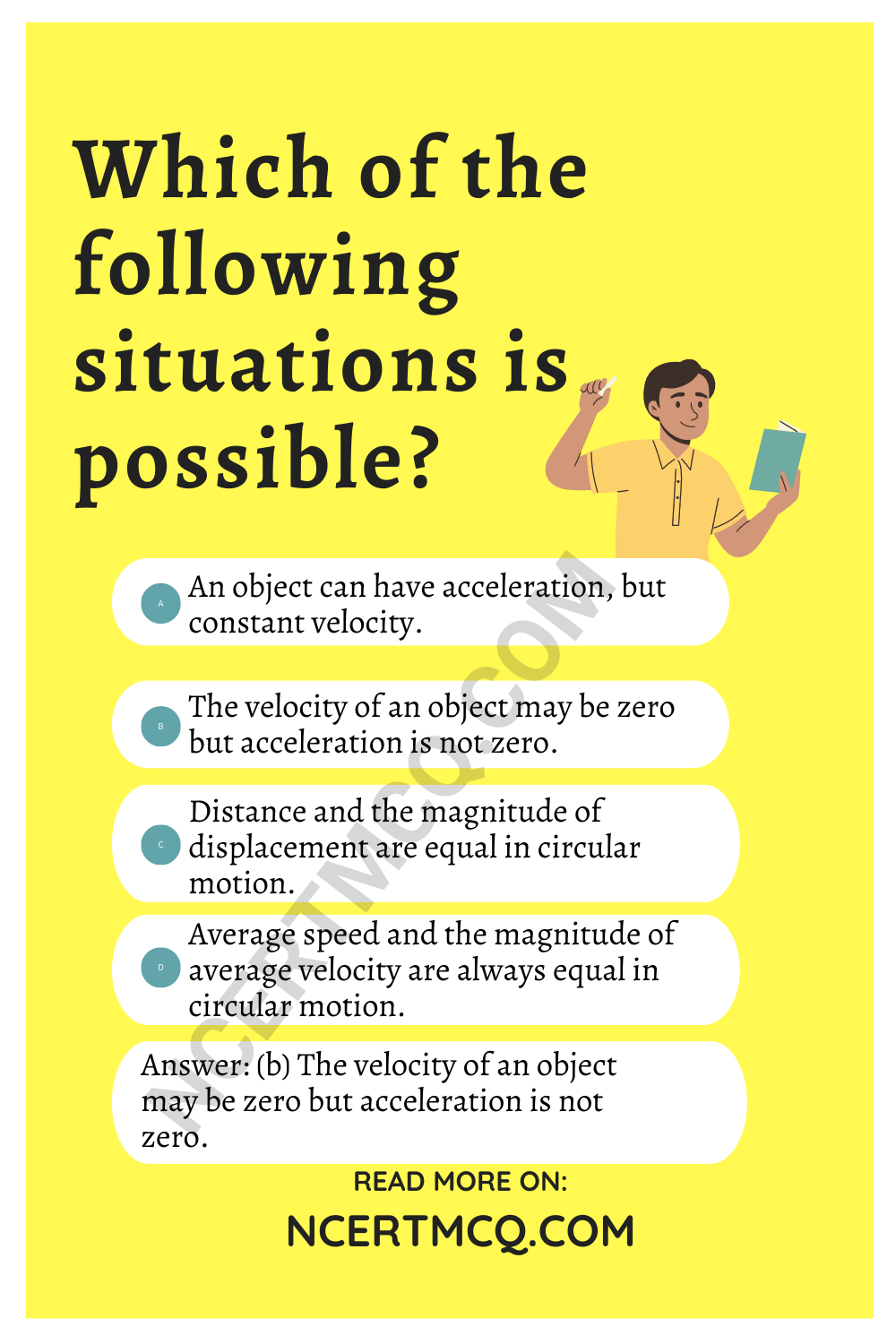Question 20.
Which of the following graphs is not possible?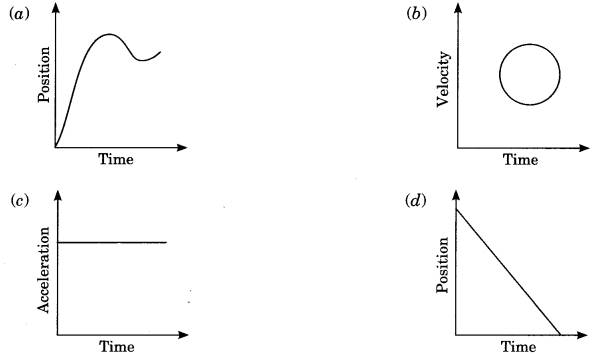Fill in the blanks:

1. The total path length travelled by a body in a given interval of time is called …………….

2. A body moving in a straight line has a uniform motion if it travels ……………. distance in …………… intervals of time.

3. Velocity is defined as ……………. per unit time.

4. Speed is scalar quantity and velocity is ……………… quantity.

5. If speed of a body is continuously decreasing, the body is said to ……………

6. Acceleration is vector quantity and its SI unit is ……………..

7. A physical quantity that has magnitude as well as …………… is called vector quantity.

8. The slope of velocity-time graph gives …………….. and the slope of displacement-time graph gives ……………..

III. Match the following columns

 Column I Column II (a) Straight line parallel to time axis in velocity-time graph (i) Scalar quantity (b) Distance (ii) Body at rest (c) Displacement (iii) Motion of object in circular path with uniform speed (d) Straight line parallel to time axis in position time graph (iv) Body in uniform motion (e) Uniform circular motion (v) Rate of change of velocity with respect to time (f) Slope of velocity-time graph gives (vi) Shortest distance between initial and final position (g) Acceleration (vii) Acceleration

 Column I Column II (a) Straight line parallel to time axis in velocity-time graph (i) Scalar quantity (b) Distance (ii) Body at rest (c) Displacement (iii) Motion of object in circular path with uniform speed (d) Straight line parallel to time axis in position time graph (iv) Body in uniform motion (e) Uniform circular motion (v) Rate of change of velocity with respect to time (f) Slope of velocity-time graph gives (vi) Shortest distance between initial and final position (g) Acceleration (vii) Acceleration

Complete the crossword given below: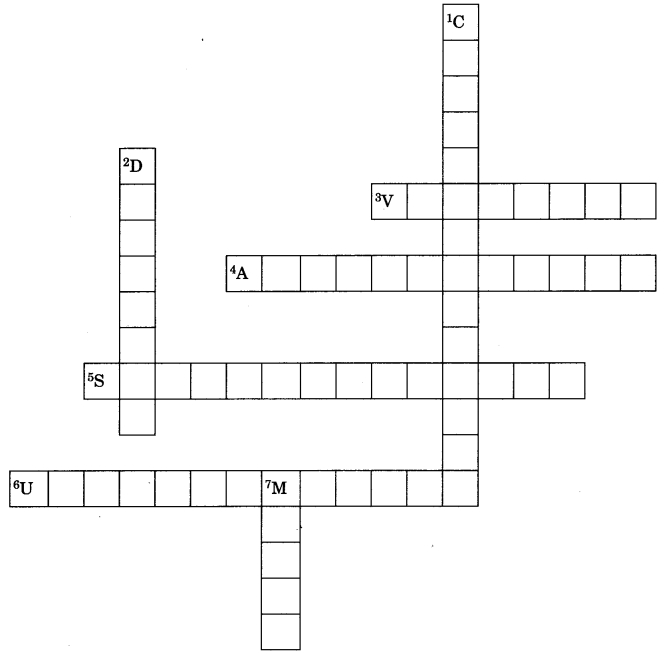Across:
3. Displacement per unit time
4. Rate of change of velocity
5. A physical quantity which is described completely by its magnitude only
6. If a body travels equal distance in equal intervals of time in straight line then motion is
Down:
1. Motion of body in circular path
2. Area of speed-time graph gives
7. SI unit of distance and displacement